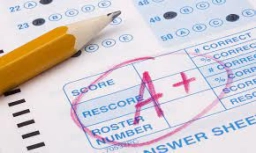# Five-minute 80951

Karel has an average grade of exactly 1.12 from five-minute episodes. Prove that at least 22 of them have one.

z = 22*1+3*2
z2 = 23*1+1*2+1*3

### Step-by-step explanation:Did you find an error or inaccuracy? Feel free to write us. Thank you!

Tips for related online calculators
Looking for help with calculating arithmetic mean?
Looking for a statistical calculator?
Do you solve Diofant problems and looking for a calculator of Diofant integer equations?
Do you have a linear equation or system of equations and looking for its solution? Or do you have a quadratic equation?

#### Grade of the word problem:

We encourage you to watch this tutorial video on this math problem: